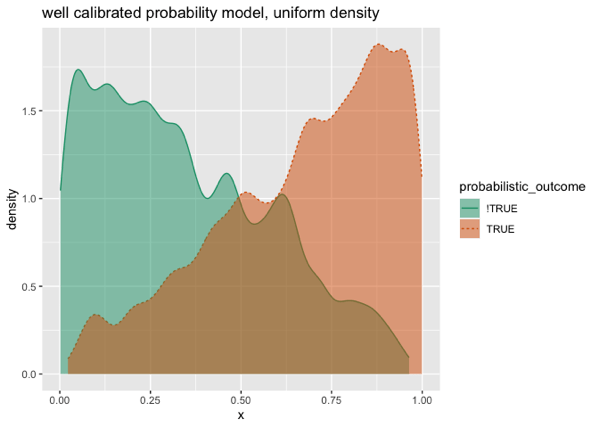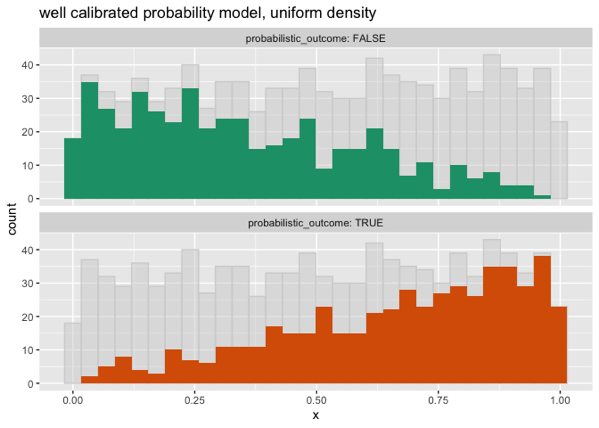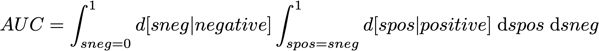# 0.83 is a Special AUC

0.83 (or more precisely 5/6) is a special Area Under the Curve (AUC), which we will show in this note.

For a classification problem a good probability model has two important properties:

1. The model is well calibrated. When the model says there is a p-probability of being in the class, the item is in the class with a frequency close to p.
2. The model is useful, or is a strong signal. It doesn’t place most of its predictions near a constant such as the training prevalence.

In general good probability models are much more useful that mere classification rules (for some notes on this, please see here).

An ideal model would always return a score of zero or one, and always be right (items with a score of zero never being in the class, and items with a score of one always being in the class). Of course, this is unlikely to be achieved for real world problems.

Now let’s consider a model that is perfectly calibrated, but only somewhat useful. Instead of the model scores being concentrated near zero and one, they are uniformly distributed in the interval between zero and one. Let’s also assume our class prevalence is 0.5.

This model has a decent looking Receiver Operating Characteristic (ROC) plot, as we can see using R.

```library(WVPlots)

d_uniform <- data.frame(x = runif(1000))
d_uniform\$probabilistic_outcome <- d_uniform\$x >= runif(nrow(d_uniform))

ROCPlot(
d_uniform,
'x',
'probabilistic_outcome',
truthTarget = TRUE,
title = 'well calibrated probability model, uniform density')
```In the limit the Area Under the Curve (AUC) of this ROC plot is going to converge to: 5/6 or about 0.83, which we will derive later.

Slowing down this plot a bit is useful].

```ThresholdPlot(
d_uniform,
'x',
'probabilistic_outcome', truth_target = TRUE,
title = 'well calibrated probability model, uniform density')
``````DoubleDensityPlot(
d_uniform,
'x',
'probabilistic_outcome', truth_target = TRUE,
title = 'well calibrated probability model, uniform density')
``````ShadowHist(
d_uniform,
'x',
'probabilistic_outcome',
title = 'well calibrated probability model, uniform density')
```Back to the AUC.

One interpretation of the AUC is: it is how often a uniformly selected positive example gets a higher score than a uniformly selected negative example (for example, please see here). So we are interested in the probability densities `d[score|positive]` and `d[score|negative]`. By Bayes’ Law we have

```  d[score|positive] = P[postive|score] d[score] / P[positive]
=       score          1    /   (1 / 2)
= 2 * score

d[score|negative] = P[negative|score] d[score] / P[negative]
=     (1 - score)      1     /  (1 / 2)
= 2 * (1 - score)
```

(In the above the `d[score] = 1` is because `score` is uniformly distributed in the unit interval, and we are only claiming this relation for scores in the unit interval. The `P[positive] = P[negative] = 1/2` is from our prevalence 1/2 assumption.)

So we are interested in the area of where a score of a negative example `sneg` is no more than the score of a positive example `spos`. This is the following nested integral.We substitute in our formulas for the conditional densities to get:And we finish the calculation in Python/sympy.

```from sympy import *

spos, sneg = symbols('spos sneg')

integrate(
2 * (1 - sneg) * integrate(2 * spos,
(spos, sneg, 1)),
(sneg, 0, 1))

# 5/6
```

And we get the claimed 5/6, which is about 0.83.

Categories: Tutorials### jmount

Data Scientist and trainer at Win Vector LLC. One of the authors of Practical Data Science with R.